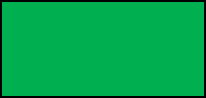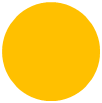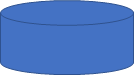# What is a Polygon | Polygon Examples

What is a Polygon?

Polygon is :

•  A figure made up of only straight line segments as their sides.
• Have three or more than three sides.

### Polygon Examples

Example 1

State whether the given figure, a polygon ?Explanation:

Polygon is :

· A figure made up of only straight line segments as their sides.

· Have three or more than three sides.Given figure is made up of only straight line segments and has three sides.

Hence, it is a polygon.

Example 2

State whether the given figure, a polygon?Explanation:

Polygon is :

· A figure made up of only straight line segments as their sides.

· Have three or more than three sides.Given figure is made up of only straight line segments and has four sides.

Hence, it is a polygon.

Example 3

State whether the given figure, a polygon ?Explanation:

Polygon is :

·  A figure made up of only straight line segments as their sides.

·  Have three or more than three sides.Given figure is made up of curved lines.

Hence, it is not a polygon.

Example 4

State whether the given figure, a polygon?Explanation:

Polygon is :

· A figure made up of only straight line segments as their sides.

· Have three or more than three sides.Given figure is made up of curved lines.

Hence, it is not a polygon.